# Texas Go Math Grade 5 Lesson 2.6 Answer Key Divide by 2-Digit Divisors

Refer to our Texas Go Math Grade 5 Answer Key Pdf to score good marks in the exams. Test yourself by practicing the problems from Texas Go Math Grade 5 Lesson 2.6 Answer Key Divide by 2-Digit Divisors.

## Texas Go Math Grade 5 Lesson 2.6 Answer Key Divide by 2-Digit Divisors

Unlock the Problem

Mr. Yates owns a smoothie shop. To mix a batch of his famous smoothies, he uses 18 ounces of orange juice. Each day he uses 560 ounces of orange juice. how many batches of smoothies can Mr. Yates make in a day?

• Underline the sentence that tells you what you are trying to find.
• Circle the numbers you need to use.

Divide. 560 ÷ 18
Estimate. 540 ÷ 18 = 30

STEP 1: Use the estimate to place the first digit in the quotient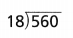The first digit of the quotient will be in the ten’s place.

STEP 2: Divide the tens.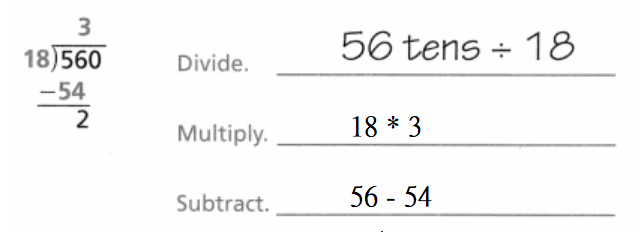Check. 2 tens cannot be shared among 18 groups without regrouping.

STEP 3: Divide the ones.Since 31 is close to the estimate of 30, the answer is reasonable.
So, Mr. Yates can make 30 batches of smoothies each day.

Math Talk
Mathematical Processes

Explain what the remainder 2 represents.
The remainder “2” represents the number of cups of orange juice after the number of batches of smoothies Mr. Yates make in a day

Example

Every Wednesday, Mr. Yates orders fruit. He has set aside $1,250 to purchase Valencia oranges. Each box of Valencia oranges costs$41.
How many boxes of Valencia oranges can Mr. Yates purchase?
It is given that
Every Wednesday, Mr. Yates orders fruit. He has set aside $1,250 to purchase Valencia oranges. Each box of Valencia oranges costs$41.
So,
The number of boxes of Valencia oranges can Mr. yates purchase = $$\frac{1,250}{41}$$
= 30.48
≅ 31 boxes

Divide. 1,250 ÷ 41So, Mr. Yates can buy 30 boxes of Valencia oranges.

Share and Show

Question 1.The given division expression is: 620 ÷ 28
Now,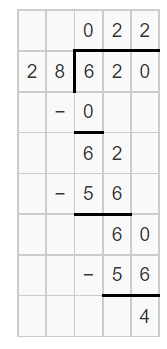Hence, from the above,
We can conclude that
620 ÷ 28 = 22 R 4

Question 2.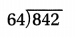The given division expression is: 842 ÷ 64
Now,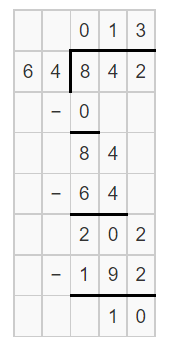Hence, from the above,
We can conclude that
842 ÷ 64 = 13 R 10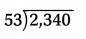The given division expression is: 2,340 ÷ 53
Now,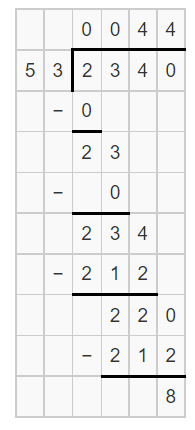Hence, from the above,
We can conclude that
2,340 ÷ 53 = 44 R 8

Question 4.
723 ÷ 31
The given division expression is: 723 ÷ 31
Now,Hence, from the above,
We can conclude that
723 ÷ 31 = 23 R 10

Question 5.
1,359 ÷ 45
The given division expression is: 1,359 ÷ 45
Now,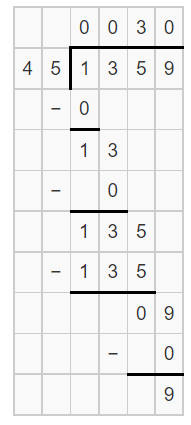Hence, from the above,
We can conclude that
1,359 ÷ 45 = 30 R 9

Question 6.
7,925 ÷ 72
The given division expression is: 7,925 ÷ 72
Now,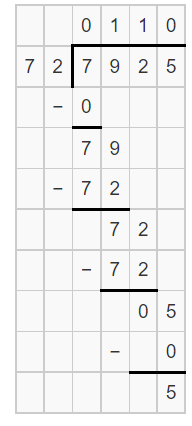Hence, from the above,
We can conclude that
7,925 ÷ 72 = 110 R 5

Problem Solving

Question 7.
775 ÷ 35
The given division expression is: 775 ÷ 35
Now,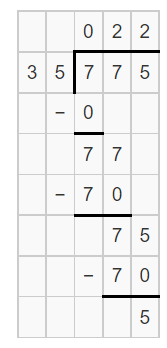Hence, from the above,
We can conclude that
775 ÷ 35 = 22 R 5

Question 8.
820 ÷ 41
The given division expression is: 820 ÷ 41
Now,Hence, from the above,
We can conclude that
820 ÷ 41 = 20

805 ÷ 24
The given division expression is: 805 ÷ 24
Now,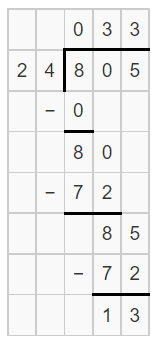Hence, from the above,
We can conclude that
805 ÷ 24 = 33 R 13

Question 10.
1,166 ÷ 53
The given division expression is: 1,166 ÷ 53
Now,Hence, from the above,
We can conclude that
1,166 ÷ 53 = 22

Question 11.
1,989 ÷ 15
The given division expression is: 1,989 ÷ 15
Now,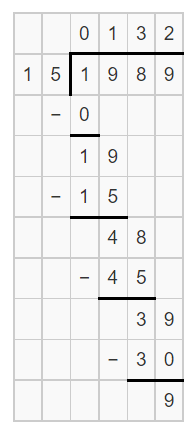Hence, from the above,
We can conclude that
1,989 ÷ 15 = 132 R 9

Question 12.
3,927 ÷ 35
The given division expression is: 3,927 ÷ 35
Now,Hence, from the above,
We can conclude that
3,927 ÷ 35 = 112 R 7

Question 13.
Why can you use multiplication to check division? Explain.
Division and multiplication are inverse or opposite operations. You can use multiplication to check your division answer this way. If there is a remainder, add it to the multiplication product. Compare this answer to the dividend

Problem Solving

Use the list at the right to solve 14-16.Question 14.
A smoothie shop receives delivery of 980 ounces of grape juice. How many Royal Purple smoothies can be made with grape juice?
It is given that
A smoothie shop receives delivery of 980 ounces of grape juice
Now,
The given information is:Now,
From the above,
We can observe that
To make the Royal Purple Smoothie,
We need 22 ounces of grape juice
So,
The number of Royal Purple smoothies can be made with grape juice = (The total number of ounces of grape juice) ÷ (The number of ounces of grape juice in the Royal Purple Smoothie)
= $$\frac{980}{22}$$
Now,
By using the Long Division,Hence, from the above,
We can conclude that
The number of Royal Purple smoothies that can be made with grape juice is: 44 Royal Purple Smoothies

H.O.T. Multi-Step The shop has 1,260 ounces of cranberry juice and 650 ounces of passion fruit juice. If the juices are used to make Crazy Cranberry smoothies, which juice will run out first? How much of the other juice will be left?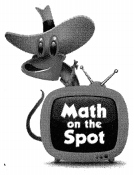It is given that
The shop has 1,260 ounces of cranberry juice and 650 ounces of passion fruit juice.
Now,
The given information is:Now,
From the above,
We can observe that
In Cranberry Smoothie,
The number of ounces of cranberry juice is: 20 ounces
The number of ounces of passion fruit juice is: 10 ounces
Now,
The total number of ounces of cranberry juice = $$\frac{1,260}{20}$$
= 63 ounces
The total number of ounces of passion fruit juice = $$\frac{650}{10}$$
= 65 ounces
So,
The total number of ounces that is leftover = 65 – 63
= 2 ounces
So,
63 ounces < 65 ounces
Hence, from the above,
We can conclude that
The cranberry juice will run out first
The amount of the other juice that will be leftover is: 2 ounces

Question 16.
H.O.T. In the refrigerator, there are 680 ounces of orange juice and 410 ounces of mango juice. How many Orange Tango smoothies can be made? Explain your reasoning.
It is given that
In the refrigerator, there are 680 ounces of orange juice and 410 ounces of mango juice
Now,
The given information is:Now,
From the above,
We can observe that
In Orange Tango Smoothie,
The number of ounces of orange juice is: 18 ounces
The number of ounces of mango juice = 12 ounces
So,
The number of Orange Tango smoothies that can be made = $$\frac{680 + 410}{18 + 12}$$
= $$\frac{1,090}{30}$$
Now,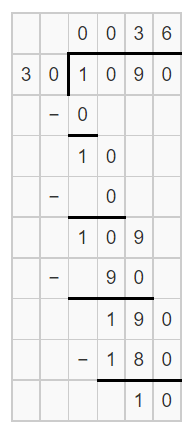Hence, from the above,
We can conclude that
The number of Orange Tango smoothies that can be made is: 36 Smoothies

Question 17.
A shipment of 572 scented erasers has arrived at the school store. You want to sell them in sets of 15. How many full sets of erasers can you make?
(A) 40
(B) 38
(C) 39
(D) 37
It is given that
A shipment of 572 scented erasers has arrived at the school store. You want to sell them in sets of 15.
Now,
According to the given information,
The number of full sets of erasers you can make = $$\frac{572}{15}$$
Now,Hence, from the above,
We can conclude that
The number of full sets of erasers you can make is:Question 18.
Darius counted that he walks 864 steps from his house to the bookstore. If Darius takes 52 steps each minute, how long will it take him to reach the bookstore?
(A) 31 minutes
(B) 17 minutes
(C) 13 minutes
(D) 20 minutes
It is given that
Darius counted that he walks 864 steps from his house to the bookstore. and Darius takes 52 steps each minute
So,
The amount of time it took Darius to reach the bookstore = $$\frac{864}{52}$$
Now,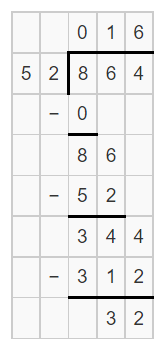Hence, from the above,
We can conclude that
The amount of time it took Darius to reach the bookstore is:Question 19.
Multi-Step The school store has 1,262 bouncy balls. The bouncy balls are packaged in sets. Any single bouncy ball is sold for $1. If all the bouncy balls sell, which of the following will give the store the best profit? (A) Sets of 25 for$13
(B) Sets of 16 for $9 (C) Sets of 12 for$7
(D) Sets of 10 for \$6

Texas Test Prep

Question 20.
James has 1,836 marbles. He decides to divide them equally among 23 boxes. How many marbles will James have leftover?
(A) 19
(B) 23
(C) 90
(D) 79
It is given that
James has 1,836 marbles. He decides to divide them equally among 23 boxes
Now,
The number of marbles in each box = 1,836 ÷ 23
Now,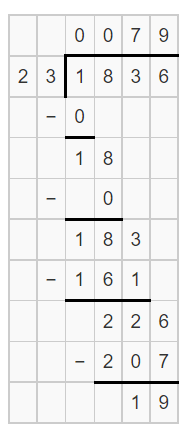So,
The number of marbles that are leftover is: 19
Hence, from the above,
We can conclude that
The number of marbles will James have leftover is: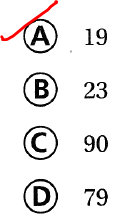### Texas Go Math Grade 5 Lesson 2.6 Homework and Practice Answer Key

Question 1.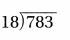The given division expression is: 783 ÷ 18
Now,
By using the Long Division,Hence, from the above,
We can conclude that
783 ÷ 18 = 43 R 9

Question 2.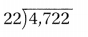The given division expression is: 4,722 ÷ 22
Now,
By using the Long Division,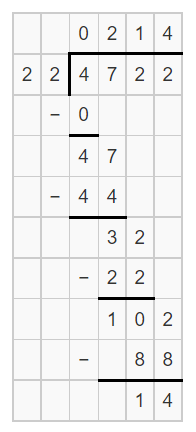Hence, from the above,
We can conclude that
4,722 ÷ 22 = 214 R 14

Question 3.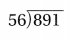The given division expression is: 891 ÷ 56
Now,
By using the Long Division,Hence, from the above,
We can conclude that
891 ÷ 56 = 15 R 51

Question 4.The given division expression is: 3,192 ÷ 19
Now,
By using the Long Division,Hence, from the above,
We can conclude that
3,192 ÷ 19 = 168

Question 5.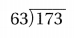The given division expression is: 173 ÷ 63
Now,
By using the Long Division,Hence, from the above,
We can conclude that
173 ÷ 63 = 2 R 47

Go Math Answer Key Grade 5 Practice and Homework Lesson 2.6 Question 6.The given division expression is: 8,382 ÷ 43
Now,
By using the Long Division,Hence, from the above,
We can conclude that
8,382 ÷ 43 = 192 R 40

Question 7.The given division expression is: 5,109 ÷ 39
Now,
By using the Long Division,Hence, from the above,
We can conclude that
5,109 ÷ 39 = 131

Question 8.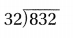The given division expression is: 832 ÷ 32
Now,
By using the Long Division,Hence, from the above,
We can conclude that
832 ÷ 32 = 26

Question 9.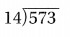The given division expression is: 573 ÷ 14
Now,
By using the Long Division,Hence, from the above,
We can conclude that
573 ÷ 14 = 40 R 13

Explain.

Question 10.
You can check your answer to a division problem by using multiplication. How can you check the answer when a division problem has a remainder?
When a math problem has a remainder, it just means that there is a number left over after the division is done. To check your answer, just multiply your quotient and divisor and add your remainder. Your total should match the number you started with.

Problem Solving

Question 11.
Leon has 1,353 bottle caps in his collection. He decides to divide them evenly among 47 boxes. How many bottle caps will Leon have leftover?
It is given that
Leon has 1,353 bottle caps in his collection. He decides to divide them evenly among 47 boxes
So,
According to the given information,
The number of bottle caps will Leon have = $$\frac{1,353}{47}$$
Now,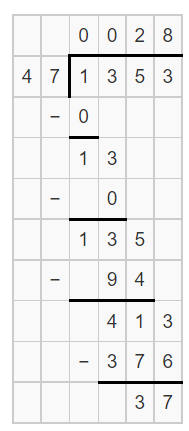Now,
We know that,
The remainder will represent the “Leftovers”
Hence, from the above,
We can conclude that
The number of bottle caps will Leon have leftover is: 37 bottle caps

Question 12.
It takes 48 ounces of vegetable broth to make a pot of Chef Zoe’s minestrone soup. How many pots of soup can be made with 432 ounces of broth?
It is given that
It takes 48 ounces of vegetable broth to make a pot of Chef Zoe’s minestrone soup
So,
According to the given information,
The number of pots of soup that can be made from 432 ounces of broth = $$\frac{432}{48}$$
Now,Hence, from the above,
We can conclude that
The number of pots of soup that can be made from 432 ounces of broth is: 9 pots of soup

Lesson Check

Question 13.
Tamera has a rubber band ball made of 3,241 rubber bands. How many complete smaller rubber band balls can she make if each small ball is made of 80 bands?
(A) 28
(B) 40
(C) 53
(D) 41
It is given that
Tamera has a rubber band ball made of 3,241 rubber bands and each small ball is made of 80 bands
So,
According to the given information,
The number of complete smaller rubber band balls Tamera can make = $$\frac{3,241}{80}$$
Now,Hence, from the above,
We can conclude that
The number of complete smaller rubber band balls Tamera can make is:Question 14.
Sam picks out a book that has 468 pages. He wants to finish the book in 36 days. If he reads the same number of pages each day, how many pages should he read?
(A) 23
(B) 11
(C) 13
(D) 11
It is given that
Sam picks out a book that has 468 pages. He wants to finish the book in 36 days
S0,
According to the given information,
The number of pages Sam should read = $$\frac{468}{36}$$
Now,Hence, from the above,
We can conclude that
The number of pages Sam should read is: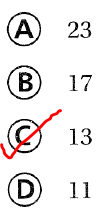Question 15.
A baker has 834 fluid ounces of muffin batter. Each muffin needs 12 fluid ounces of batter. How many muffins can the baker make?
(A) 70
(B) 69
(C) 68
(D) 65
It is given that
A baker has 834 fluid ounces of muffin batter. Each muffin needs 12 fluid ounces of batter
So,
According to the given information,
The number of muffins the baker can make = $$\frac{834}{12}$$
Now,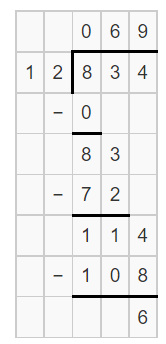Hence, from the above,
We can conclude that
The number of muffins the baker can make is:Question 16.
Springfield has an aquarium that holds 336 gallons of water. It takes 24 hours to fill the aquarium. How many gallons of water fill the aquarium each hour?
(A) 16 gallons
(B) 14 gallons
(C) 15 gallons
(D) 13 gallons
It is given that
Springfield has an aquarium that holds 336 gallons of water. It takes 24 hours to fill the aquarium.
So,
According to the given information,
The number of gallons of water fill the aquarium each hour = $$\frac{336}{24}$$
Now,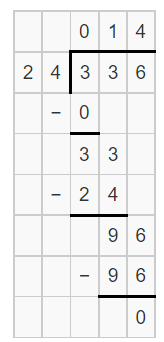Hence, from the above,
We can conclude that
The number of gallons of water fill the aquarium each hour is: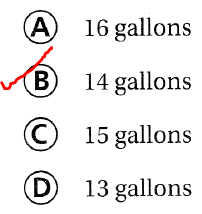Question 17.
Multi-Step A juice bar has 768 ounces of passion fruit juice and 810 ounces of berry juice. Passion fruit juice is sold in 16-ounce cups. Berry juice is sold in 18-ounce cups. Which statement is true? ‘
(A) The juice bar can sell 3 more cups of berry juice than passion fruit juice.
(B) The juice bar can sell the same number of cups of berry juice and passion fruit juice.
(C) The juice bar can sell fewer than 90 total cups of berry juice and passion fruit juice.
(D) The juice bar can sell 3 more cups of passion fruit juice than berry juice.
It is given that
A juice bar has 768 ounces of passion fruit juice and 810 ounces of berry juice. Passion fruit juice is sold in 16-ounce cups. Berry juice is sold in 18-ounce cups
So,
According to the given information,
The number of ounces of passion fruit juice = $$\frac{768}{16}$$
= 48
The number of ounces of Berry juice = $$\frac{810}{18}$$
= 45
Now,
The difference between the number of ounces of passion fruit juice and Berry juice = 48 – 45 = 3 ounces
Hence, from the above,
We can conclude that
The statement that is true is:Question 18.
Multi-Step A city has 7,204 recycling bins. The city gives half of the recycle bins to its citizens. The rest of the recycle bins are divided into 23 equal groups for city parks. How many recycle bins are left over?
(A) 14
(B) 156
(C) 138
(D) 28
It is given that
A city has 7,204 recycling bins. The city gives half of the recycle bins to its citizens. The rest of the recycle bins are divided into 23 equal groups for city parks
So,
According to the given information,
The number of recycle bins that are leftover = $$\frac{7,204 ÷ 2}{23}$$
= $$\frac{3,602}{23}$$
Now,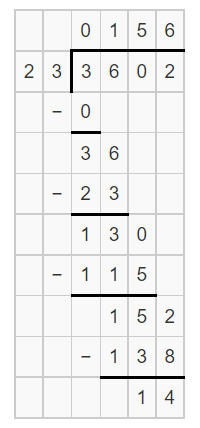Hence, from the above,
We can conclude that
The number of recycle bins that are leftover is: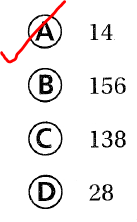Scroll to Top